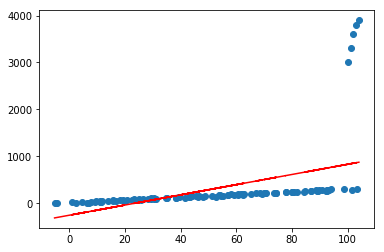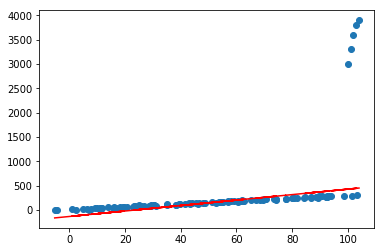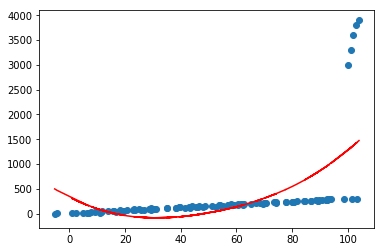$$y(x,w)=w_0+w_1x_1+\cdots+w_Dx_D$$

### 一.线性基函数模型¶

$$y(x,w)=w_0+\sum_{j=1}^{M-1}w_j\phi_j(x)$$

$$y(x,w)=w^T\phi(x)$$

$\phi_j(\cdot)$除了我们自定义一些复杂的函数外，还有一些常用的基函数，比如

#### 多项式基函数¶

$$\phi_j(x)=x^j$$

#### 高斯基函数¶

$$\phi_j(x)=exp\left \{-\frac{(x-\mu_j)^2}{2s^2}\right \}$$

#### sigmoid基函数¶

$$\phi_j(x)=\sigma\left( \frac{x-\mu_j}{s}\right )$$

### 二.最小平方和误差与高斯极大似然估计¶

$$t=y(w,x)+\epsilon$$

$$p(t\mid x,w,\beta)=N(t\mid y(x,w),\beta^{-1})$$

$$E[t\mid x]=\int tp(t\mid)dt=y(x,w)$$

$$p(t\mid X,w,\beta)=\prod_{n=1}^N N(t_n\mid w^T\phi(x_n),\beta^{-1})$$

$$ln\ p(t\mid w,\beta)=\sum_{n=1}^Nln\ N(t_n\mid w^T\phi(x_n),\beta^{-1})\\ =\frac{N}{2}ln\ \beta-\frac{N}{2}ln(2\pi)-\beta E_D(w)$$

$$E_D(w)=\frac{1}{2}\sum_{n=1}^N(t_n-w^T\phi(x_n))^2$$

$$w_{ML}=(\Phi^T\Phi)^{-1}\Phi^Tt$$

$$\Phi=\begin{pmatrix} \phi_0(x_1) &\phi_1(x_1) & \cdots &\phi_{M-1}(x_1) \\ \phi_0(x_2) & \phi_1(x_2) & \cdots & \phi_{M-1}(x_2)\\ \vdots & \vdots &\ddots &\vdots \\ \phi_0(x_N) &\phi_1(x_N) & \cdots & \phi_{M-1}(x_N) \end{pmatrix}$$

#### $w_0$的最优解¶

$$E_D(w)=\frac{1}{2}\sum_{n=1}^N(t_n-w_0-\sum_{j=1}^{M-1}w_j\phi_j(x_n))^2$$

$$w_0=\bar{t}-\sum_{j=1}^{M-1}w_j\bar{\phi_j}$$

$$\bar{t}=\frac{1}{N}\sum_{n=1}^Nt_n,\bar{\phi_j}=\frac{1}{N}\sum_{n=1}^N\phi_j(x_n)$$

#### $\beta$的最优解¶

$$\frac{1}{\beta_{ML}}=\frac{2}{N}E_D(w_{ML})$$

### 三.$L_2$正则化与最大后验估计¶

$$p(w)=N(w\mid m_0,S_0)$$

$$p(w\mid t)\propto p(w)p(t\mid w)$$

$$p(w\mid t)=N(w\mid m_N,S_N)$$

$$m_N=S_N(S_0^{-1}m_0+\beta\Phi^Tt)\\ S_N^{-1}=S_0^{-1}+\beta\Phi^T\Phi$$

$$m_N=\beta S_N\Phi^Tt\\ S_N^{-1}=\alpha I+\beta\Phi^T\Phi$$

$$ln\ p(w\mid t)=-\frac{\beta}{2}\sum_{n=1}^N\left\{ t_n-w^T\phi(x_n) \right\}^2-\frac{\alpha}{2}w^Tw+const$$

### 四.贝叶斯估计¶

$$p(\hat{t}\mid \hat{x},X,t,\alpha,\beta)=\int p(\hat{t}\mid w,\beta,\hat{x})p(w\mid X,t,\alpha,\beta)dw$$

$$p(w\mid X,t,\alpha,\beta)=N(w\mid m_N,S_N)$$

$$p(\hat{t}\mid \hat{x},w,\beta)=N(\hat{t}\mid y(\hat{x},w),\beta^{-1})\\ =N(\hat{t}\mid w^T\Phi(\hat{x}),\beta^{-1})$$

$$p(\hat{t}\mid \hat{x},X,t,\alpha,\beta)=N(\hat{t}\mid m_N^T\phi(\hat{x}),\sigma_N^2(\hat{x})))$$

$$\sigma_N^2(\hat{x})=\frac{1}{\beta}+\phi(\hat{x})^TS_N\phi(\hat{x})$$

$$\hat{y}(w,\hat{x})=m_N^T\phi(\hat{x})$$

$$w=m_N$$

### 五.代码实现¶

In :
%matplotlib inline

In :
"""

"""
import numpy as np
import matplotlib.pyplot as plt

class LinearRegression(object):
def __init__(self, basis_func=None, alpha=1, beta=1):
"""
:param basis_func: list,基函数列表，包括rbf,sigmoid,poly_{num},linear，默认None为linear，其中poly_{num}中的{num}表示多项式的最高阶数
:param alpha: alpha/beta表示理解为L2正则化项的大小，默认为1
:param beta: 噪声，默认为1
"""
if basis_func is None:
self.basis_func = ['linear']
else:
self.basis_func = basis_func
self.alpha = alpha
self.beta = beta
# 特征均值、标准差
self.feature_mean = None
self.feature_std = None
# 训练参数
self.w = None

def _map_basis(self, X):
"""
将X进行基函数映射
:param X:
:return:
"""
x_list = []
for basis_func in self.basis_func:
if basis_func == "linear":
x_list.append(X)
elif basis_func == "rbf":
x_list.append(np.exp(-0.5 * X * X))
elif basis_func == "sigmoid":
x_list.append(1 / (1 + np.exp(-1 * X)))
elif basis_func.startswith("poly"):
p = int(basis_func.split("_"))
for pow in range(1, p + 1):
x_list.append(np.power(X, pow))
return np.concatenate(x_list, axis=1)

def fit(self, X, y):
self.feature_mean = np.mean(X, axis=0)
self.feature_std = np.std(X, axis=0) + 1e-8
X_ = (X - self.feature_mean) / self.feature_std
X_ = self._map_basis(X_)
X_ = np.c_[np.ones(X_.shape), X_]
self.w = self.beta * (
np.linalg.inv(self.alpha * np.eye(X_.shape) + self.beta * X_.T @ X_)) @ X_.T @ y.reshape((-1, 1))

def predict(self, X):
X_ = (X - self.feature_mean) / self.feature_std
X_ = self._map_basis(X_)
X_ = np.c_[np.ones(X_.shape), X_]
return (self.w.T @ X_.T).reshape(-1)

def plot_fit_boundary(self, x, y):
"""
绘制拟合结果
:param x:
:param y:
:return:
"""
plt.scatter(x[:, 0], y)
plt.plot(x[:, 0], self.predict(x), 'r')


### 测试¶

In :
#造伪样本
X=np.linspace(0,100,100)
X=np.c_[X,np.ones(100)]
w=np.asarray([3,2])
Y=X.dot(w)
X=X.astype('float')
Y=Y.astype('float')
X[:,0]+=np.random.normal(size=(X[:,0].shape))*3#添加噪声
Y=Y.reshape(100,1)

In :
#加噪声
X=np.concatenate([X,np.asanyarray([[100,1],[101,1],[102,1],[103,1],[104,1]])])
Y=np.concatenate([Y,np.asanyarray([,,,,])])

In :
lr=LinearRegression()
lr.fit(X[:,:-1],Y)
lr.plot_fit_boundary(X[:,:-1],Y)In :
#增加alpha防止过拟合
lr=LinearRegression(alpha=100)
lr.fit(X[:,:-1],Y)
lr.plot_fit_boundary(X[:,:-1],Y)In :
#换不同的基函数组合
lr=LinearRegression(basis_func=['poly_2','rbf'])
lr.fit(X[:,:-1],Y)
lr.plot_fit_boundary(X[:,:-1],Y)In [ ]: